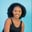Related Tags

python
python3
python programming

# How to use the NumPy.std() in PythonMaria Elijah

## Overview

NumPy is a Python library that allows us to work with numeric data. Numeric data can be created and stored in a data structure called a NumPy array. NumPy has various functions to perform calculations on the arrays of numeric data. One of these is the std() function.

The numpy. std() function finds the standard deviation of a given NumPy array along the specified axis.

### Syntax

numpy.std(
arr,
axis=None,
out=None,
overwrite_input=False,
dtype=data-type
)


### Parameters

• arr: This represents the input array.
• axis: This represents the axis on which we want to calculate the standard deviation. If the axis is 0, the direction is down the row. If it is 1, the direction is down the column.
• out: This is an optional parameter that saves the NumPy result.
• dtype: This is an optional parameter that specifies the type to use when computing the standard deviation.

### Code

The following code shows how to use the NumPy.std() function in Python:

# import numpy
import numpy as np
# create a list
my_list = [24,8,3,4,86,42,56,34,8]
# convert the list to numpy array
np_list = np.array(my_list)
# compute the std and store it
np_list_std = np.std(np_list)

print(f"The standard deviation is {np_list_std}")

### Explanation

• Line 2: We import the numpy library.
• Line 4: We create a list called my_list.
• Line 6: We convert the list to the NumPy array and store it in a variable np_list.
• Line 8: We use the np.std() function to compute the standard deviation for the np_list.
• Line 10: We display the result.

RELATED TAGS

python
python3
python programming

CONTRIBUTORMaria Elijah
RELATED COURSES

View all Courses

Keep Exploring

Learn in-demand tech skills in half the time